1- Linear classifier: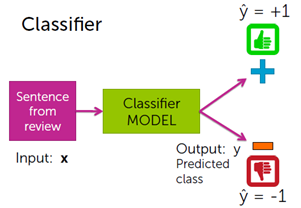A linear classifier:

• Using a training data to learn a weight or coefficient for each word.
• Calling a linear classifier, because output is weighted sum of input.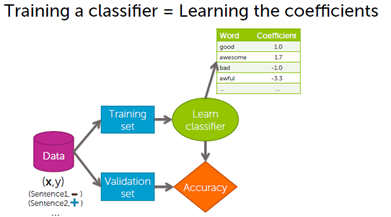Decision boundries:

Decision boundries separates positive and negative predictions:

• For linear classifiers:
• When 2 coefficients are non-zero Line
• When 3 coefficients are non-zero Plane
• When many coefficients are non-zero Hyper plane
• For more general classifiers More complicated shapes.

Linear classifier model: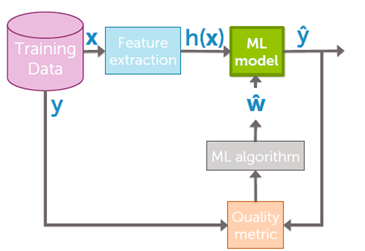a- Linear regression with one variable:

i- Model and cost function:

1-Model representation:

Our first learning algorithm will be linear regression.

More formally, in supervised learning, we have a data set and this data set is called a training set.

Let's define some notation that we're using throughout this course. We're going to define quite a lot of

symbols.

• m: to denote the number of training examples.
• x: to denote the input variables often also called the features.
• y: to denote my output variables or the target variable which i'm going to predict.
• (x,y): to denote a single training example.

To establish notation for future use, we’ll use  to denote the “input” variables (living area in this example), also called input features, and  to denote the “output” or target variable that we are trying to predict (price). A pair   is called a training example, and the dataset that we’ll be using to learn—a list of m training examples  —is called a training set. Note that the superscript “(i)” in the notation is simply an index into the training set and has nothing to do with exponentiation. We will also use X to denote the space of input values, and Y to denote the space of output values. In this example, X = Y = ℝ.

To describe the supervised learning problem slightly more formally, our goal is, given a training set, to learn a function h: X → Y so that h(x) is a “good” predictor for the corresponding value of y. For historical reasons, this function h is called a hypothesis. Seen pictorially, the process is therefore like this: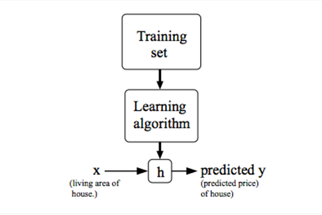When the target variable that we’re trying to predict is continuous, such as in our housing example, we call the learning problem a regression problem. When y can take on only a small number of discrete values (such as if, given the living area, we wanted to predict if a dwelling is a house or an apartment, say), we call it a classification problem.

2-Cost function:

We can measure the accuracy of our hypothesis function by using a cost function. This takes an inputs from x's and the actual output y's.To break it apart, it is  where   is the mean of the squares of   , or the difference between the predicted value and the actual value.

This function is otherwise called the "Squared error function", or "Mean squared error". The mean is halved  as a convenience for the computation of the gradient descent, as the derivative term of the square function will cancel out the.

3-Cost function - Intuition 1

If we try to think of it in visual terms, our training data set is scattered on the x-y plane. We are trying to make a straight line (defined by ) which passes through these scattered data points.

Our objective is to get the best possible line. The best possible line will be such so that the average squared vertical distances of the scattered points from the line will be the least. Ideally, the line should pass through all the points of our training data set. In such a case, the value of  will be 0. The following example shows the ideal situation where we have a cost function of 0.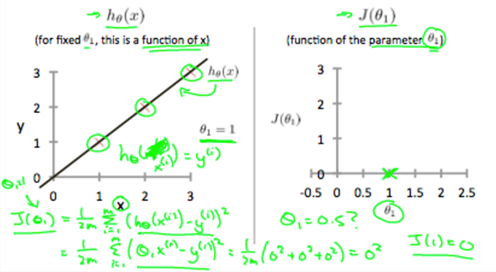When θ1=1, we get a slope of 1 which goes through every single data point in our model. Conversely, when θ1=0.5, we see the vertical distance from our fit to the data points increase.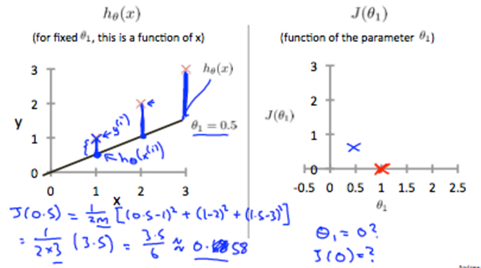This increases our cost function to 0.58. Plotting several other points yields to the following graph:

Thus as a goal, we should try to minimize the cost function. In this case, =1 is our global minimum.

ii- Parameter learning:

So we have our hypothesis function and we have a way of measuring how well it fits into the data. Now we need to estimate the parameters in the hypothesis function. That's where gradient descent comes in.

Imagine that we graph our hypothesis function based on its fields  and  (actually we are graphing the cost function as a function of the parameter estimates). We are not graphing x and y itself, but the parameter range of our hypothesis function and the cost resulting from selecting a particular set of parameters.

We put  on the x axis and  on the y axis, with the cost function on the vertical z axis. The points on our graph will be the result of the cost function using our hypothesis with those specific theta parameters. The graph below depicts such a setup.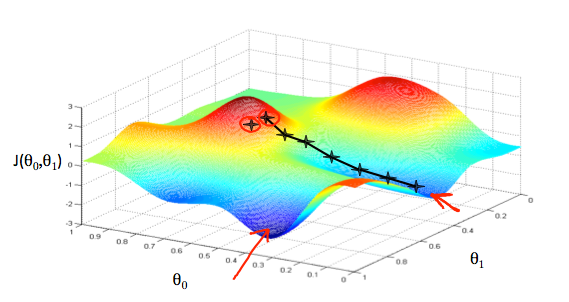We will know that we have succeeded when our cost function is at the very bottom of the pits in our graph, i.e. when its value is the minimum. The red arrows show the minimum points in the graph.

The way we do this is by taking the derivative (the tangential line to a function) of our cost function. The slope of the tangent is the derivative at that point and it will give us a direction to move towards. We make steps down the cost function in the direction with the steepest descent. The size of each step is determined by the parameter α, which is called the learning rate.

For example, the distance between each 'star' in the graph above represents a step determined by our parameter α. A smaller α would result in a smaller step and a larger α results in a larger step. The direction in which the step is taken is determined by the partial derivative of  . Depending on where one starts on the graph, one could end up at different points. The image above shows us two different starting points that end up in two different places.

repeat until convergence:Where j=0,1 represents the feature index number.

At each iteration j, one should simultaneously update the parameters  . Updating a specific parameter prior to calculating another one on the  iteration would yield to a wrong implementation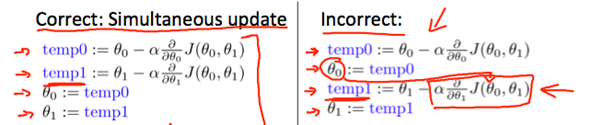In this paragraph we will explore the scenario where we use one parameter θ1 and plotte its cost function to implement a gradient descent. Our formula for a single parameter is:

Repeat until convergence:Regardless of the slope's sign foreventually converges to its minimum value. The following graph shows that when the slope is negative, the value of  increases and when it is positive, the value of  decreases.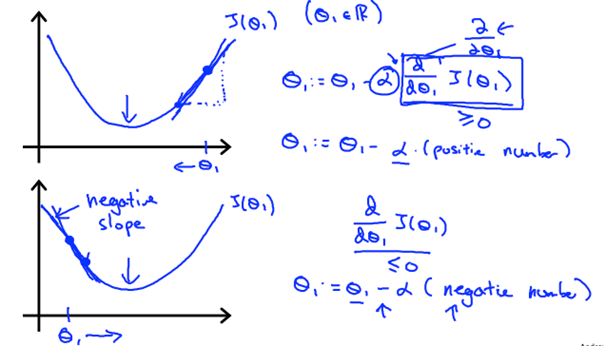On a side note, we should adjust our parameter α to ensure that the gradient descent algorithm converges in a reasonable time. Failure to converge or too much time to obtain the minimum value imply that our step size is wrong.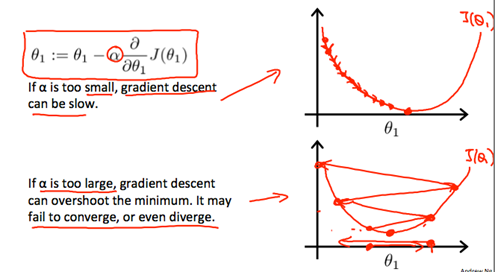• How does gradient descent converge with a fixed step size α?

The intuition behind the convergence is thatapproaches 0 as we approach the bottom of our convex function. At the minimum, the derivative will always be 0 and thus we get: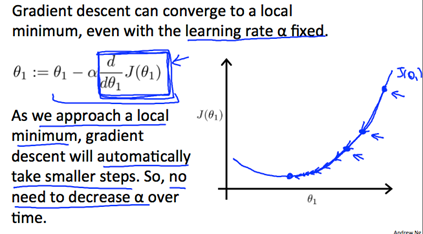3- Gradient descent for linear regression:

When specifically applied to the case of linear regression, a new form of the gradient descent equation can be derived. We can substitute our actual cost function and our actual hypothesis function and modify the equation to:Where m is the size of the training set, ​ a constant that will be changing simultaneously with θ1 and xi, yi are values of the given training set (data)

Note that we have separated out the two cases for ​θj into separate equations for θ0 and θ1; and that for θ1 we are multiplying xi at the end due to the derivative . the following is a derivation offor a single example:The point of all this is that if we start with a guess for our hypothesis and then repeatedly apply these gradient descent equations, our hypothesis will become more and more accurate.

So, this is simply gradient descent on the original cost function J. This method looks at every example in the entire training set on every step and is called batch gradient descent. Note that, while gradient descent can be susceptible to local minima in general, the optimization problem we have posed here for linear regression has only one global, and no other local, optima; thus, gradient descent always converges (assuming the learning rate α is not too large) to the global minimum. Indeed, J is a convex quadratic function. Here is an example of gradient descent as it is run to minimize a quadratic function.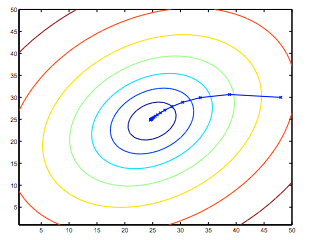The ellipses shown above are the contours of a quadratic function. Also shown is the trajectory taken by gradient descent, which was initialized at (48,30). The x’s in the figure (joined by straight lines) mark the successive values of θ that gradient descent went through as it converged to its minimum.

b- Linear regression with multiple variables:

i- Multivariate linear regression:

1-Multiple features:

Linear regression with multiple variables is also known as "multivariate linear regression". We now introduce notation for equations where we can have any number of input variables.The multivariable form of the hypothesis function accommodating these multiple features is as follows:In order to develop intuition about this function, we can think about θ0 as the basic price of a house, θ1 as the price per square meter, θ2 as the price per floor, etc.  x1 will be the number of square meters in the house,  x2 the number of floors, etc.

Using the definition of matrix multiplication, our multivariable hypothesis function can be concisely represented as:This is a vectorization of our hypothesis function for one training example;

Remark: Note that for convenience reasons in this course we assume .This allows us to do matrix operations with theta and x. Hence making the two vectors 'theta' and  match each other element-wise (that is, have the same number of elements: n+1).]

The training examples are stored in X row-wise. The following example shows us the reason behind setting  :As a result, you can calculate the hypothesis as a column vector of size (m x 1) with:The gradient descent equation itself is generally the same form; we just have to repeat it for our 'n' features:

In other words:

repeat until convergence: {}

The following image compares gradient descent with one variable to gradient descent with multiple variables: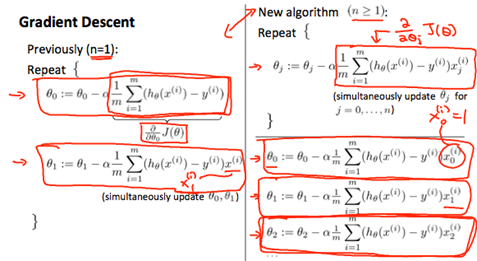3-Gradient Descent in Practice I - Feature Scaling:

We can speed up gradient descent by having each of our input values in roughly the same range. This is because θ will descend quickly on small ranges and slowly on large ranges, and so will oscillate inefficiently down to the optimum when the variables are very uneven.

The way to prevent this is to modify the ranges of our input variables so that they are all roughly the same. Ideally:

−1 ≤ x(i) ≤ 1

or

−0.5 ≤ x(i) ≤ 0.5

These aren't exact requirements; we are only trying to speed things up. The goal is to get all input variables into roughly one of these ranges, give or take a few.

Two techniques to help with this are feature scaling and mean normalization. Feature scaling involves dividing the input values by the range (i.e. the maximum value minus the minimum value) of the input variable, resulting in a new range of just 1. Mean normalization involves subtracting the average value for an input variable from the values for that input variable resulting in a new average value for the input variable of just zero. To implement both of these techniques, adjust your input values as shown in this formula:Where   is the average of all the values for feature (i) and si is the range of values (max - min), or si is the standard deviation.

Note that dividing by the range, or dividing by the standard deviation, give different results. The quizzes in this course use range - the programming exercises use standard deviation.  For example, if xi represents housing prices with a range of 100 to 2000 and a mean value of 1000, then4-Gradient Descent in Practice II - Learning Rate:

Debugging gradient descent. Make a plot with number of iterations on the x-axis. Now plot the cost function, J(θ) over the number of iterations of gradient descent. If J(θ) ever increases, then you probably need to decrease α.

Automatic convergence test. Declare convergence if J(θ) decreases by less than E in one iteration, where E is some small value such as 10−3. However, in practice it's difficult to choose this threshold value.